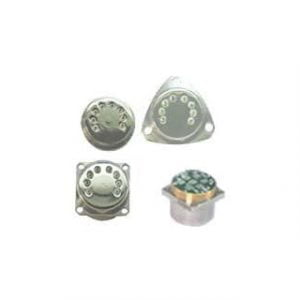Application

# How Quartz Accelerometers WorkAcceleration is a physical quantity used to reflect the speed change state of an object during motion, and it cannot be measured directly. Current acceleration sensors (accelerometers) use indirect measurement and force balance techniques to obtain the acceleration of an object. The working principle of accelerometers is based on Newton’s second law of motion: the force acting on an object is equal to the mass of the object times the acceleration. Therefore, the output of the acceleration sensor reflects the resultant force on the sensitive pendant in the sensor in the entire space, mainly including inertial force and gravity. When the object is moving in space, the sensor can obtain the acceleration of the object in real time. Integrate the accelerometer once to obtain the instantaneous velocity of the object, and then integrate the velocity to obtain the relative position of the object. Using these three parameters, you can draw the trajectory of the object.

Quartz flexible pendulum accelerometer and its development status Quartz flexible pendulum accelerometer, as a kind of force feedback pendulum accelerometer, is developed on the basis of liquid floating accelerometer. The main difference between the two is that the detection mass of the quartz flexible accelerometer is not floating, but is elastically connected to the flexible beam support, and an elastic moment is introduced, so this accelerometer has higher accuracy, strong anti-interference ability, large measurement range, and overload capacity. powerful. The performance of the flexible material directly affects the performance of the accelerometer. The materials that can be used as flexible pendulums are mainly metal and quartz. The traditional liquid-floating accelerometer pendulum uses metal materials, while the thermal expansion coefficient of quartz is much smaller than that of steel, and the material performance is better than that of metal. In addition, quartz has high fatigue resistance, and the material itself has a small lag, which is very suitable as a pendulum plate of an accelerometer. As soon as the quartz flexible accelerometer came out, it quickly replaced the liquid-floating accelerometer and became an indispensable key component in the inertial navigation and guidance system. ERICCO’s ER-QA-03A High Performance Quartz Accelerometer can carry out static test and dynamic test, which is a standard vibration sensor. It is mainly used in inertial measurement and test systems in the aerospace and aviation fields.

At present, the quartz flexible pendulum accelerometer has been widely used in the measurement of various linear acceleration and vibration acceleration, and the measurement of parameters such as speed, distance, angular velocity, and angular displacement. It has been successfully used in satellite microgravity measurement system, high-precision inertial navigation system, geotechnical foundation drilling measurement and oil drilling, continuous inclination measurement system, launch vehicle, ballistic missile, spacecraft and other military and civilian fields.